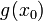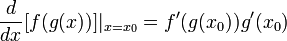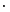# Chain rule for differentiation

This article is about a differentiation rule, i.e., a rule for differentiating a function expressed in terms of other functions whose derivatives are known.
View other differentiation rules

## Statement for two functions

Suppose$f$ and$g$ are functions such that$g$ is differentiable at a point$x = x_0$, and$f$ is differentiable at$g(x_0)$. Then the composite$f \circ g$ is differentiable at$x_0$, and we have:$\frac{d}{dx}[f(g(x))]|_{x = x_0} = f'(g(x_0))g'(x_0)$

In terms of general expressions:$\frac{d}{dx}[f(g(x))] = f'(g(x))g'(x)$

In point-free notation, we have:$(f \circ g)' = (f' \circ g) \cdot g'$

where$\cdot$ denotes the pointwise product of functions.